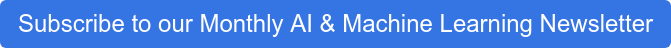## Decision Trees

As mentioned in my previous exert, I will be delving further into the complexity of the algorithm I used in my study. Following some research into decision trees and the impact they have had on healthcare and pharma I found that their presence has been assisting across the field since the early 90’s in the form of Evidence Based Medicine (EBM). The stages detailed in this process where summarised to:

• Designing a decision tree featuring all possible outcomes for a particular scenario
• Studying literature so that probabilities of each outcome can be calculated using Bayesian theorem
• Conduct sensitivity analysis (Bae, 2014)

The usefulness of the topic has been proved time and time again through providing the individual making the decision with objective evidence to mitigate the risk of misdiagnoses.

## The science behind

Decision trees fit into the supervised learning side of the machine learning algorithm classes and can be used in either regression or classification. We begin our tree with all our data at the top node, known as the root. From here we are able to perform splits on the data by creating rules for each node flowing down the tree. The algorithm will decide itself what these splits will be by estimating the potential information gain for each possibility. This process is based on how even of a divide we can get in each split and uses the calculation of entropy to perform this. The entropy score will be between zero and one, with one being the perfect score showing that the algorithm has been able to evenly split the data into two further nodes. We can continue to segregate the data into further nodes, growing the tree all the while. At this stage it is possible to over-fit the model so that it will not predict well on newly provided data. There are a few methods to prevent this, however in my efforts I decided to use pre-pruning to limit the depth of the tree prior to its creation. Once we have created our decision tree, the nodes we are left with that contain no further child nodes can be referred to as leaf nodes.

## Why This Algorithm

There are many reasons for selecting to perform machine learning via a Decision Tree. First and foremost, after creating the various levels of nodes, we can easily visualise the tree and the conditions that each split was made on. With this trait, we can say that the algorithm is a white box method of classifying. To explain this further, the decisions made by the tree are transparent to the user and can be examined at every level to gain an explanation as to why something was misclassified. From a more technical outlook, we benefit from being able to handle large diverse data sets at a relatively low computational cost. The downside of decision trees lies in their inability to classify as accurately as other algorithms, this short coming led to the like of random forest and gradient boosting being implemented.## The Data

The data available on the UCI website comes in individual comma separated files with each one representing a study on an individual. For the purpose of my experiment I joined these individual files into one. Once collated, I was left with over 75,000 rows of data each with 9 columns:

• Time in seconds
• Acceleration in G (Frontal Axis)
• Acceleration in G (Vertical Axis)
• Acceleration in G (Horizontal Axis)
• Id of mounted sensor
• Phase
• Frequency
• Label of activity (Seated on bed, Seated on chair, Lying, Ambulating)

## My Model

The purpose of the exercise I was undertaking was to create a model that could accurately classify what label of activity a person was undertaking given the data from the other columns. The predictor columns can be referred to as ‘X’ while the values to be predicted are known as ‘y’. Once I have set the X and y to the correct columns, I can remove twenty percent of my rows and save these for later as my test set. The remainder of the rows are then known as the training set.

To ensure the decision tree created was as effectively as it could be, I made use of a variety of techniques. Firstly, I implemented a grid search from sklearn’s library. Through this I will have a variety of models initialized rather than just the one. To be more specific, I'll have a model for each parameter combination and then have the best one selected. The grid search will take list of parameters, a method of scoring the model and a type of cross validation.

To explain the above in greater detail, the parameters given to the model where class weightings and maximum depth. Class weights are used to compensate for the discrepancies in activity label frequencies, I provided the model with weights for each class. By doing so the model knows how it will penalise the various classes. In my case, ambulating was the least occurring so I increased its weighting relative to the other three classes.  By providing a maximum depth, we can prevent the model from overfitting and allow it to generalise for new data provided. The method of cross validation I selected to use was the shuffle spilt technique.  This method creates distinct variations of the training set, by doing so I lessen the probability that anomalies will impact the learned model and provide a more accurate estimate of model prediction performance. The method of scoring I chose to work from was accuracy. In this case accuracy can be described as the probability that the model will correctly classify the activity that the subject is undertaking.

In my final post I will delve into the details of how I used embedpy through Kdb+ to achieve the above.